# The Spectrum object¶

RADIS has powerful tools to post-process spectra created by the line-by-line module or by other spectral codes.

At the core of the post-processing is the Spectrum class, which features methods to: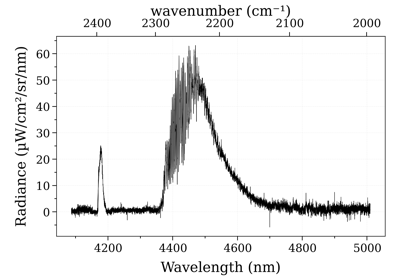Load an experimental spectrum

Load an experimental spectrumLine Survey

Line Survey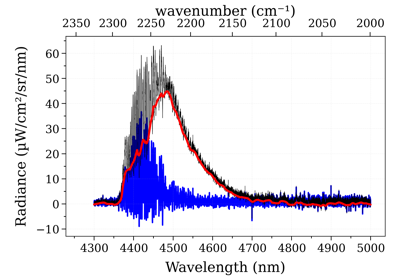Remove a baseline

Remove a baseline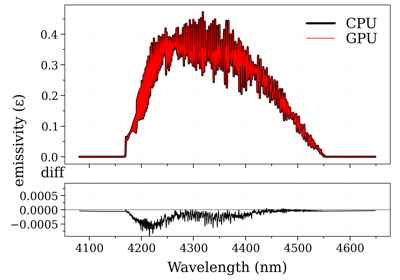GPU Accelerated Spectra

GPU Accelerated Spectra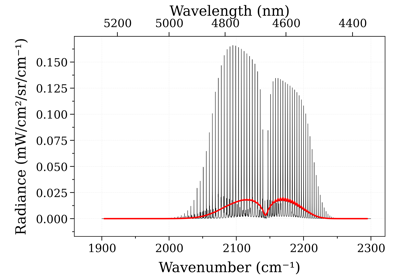Calculate non-LTE spectra of carbon-monoxide

Calculate non-LTE spectra of carbon-monoxide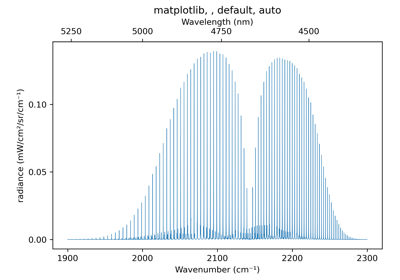Use different plot themes

Use different plot themes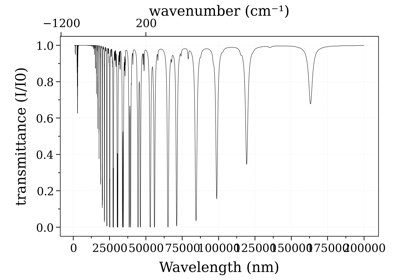Calculate a large spectrum by part

Calculate a large spectrum by partCompare CO spectrum from the GEISA and HITRAN database

Compare CO spectrum from the GEISA and HITRAN databaseCalculate a spectrum from HITEMP

Calculate a spectrum from HITEMP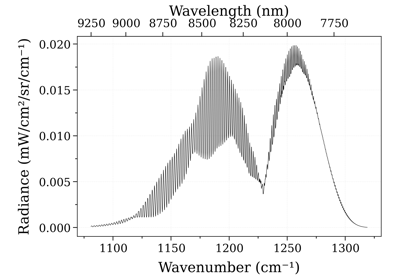Calculate a spectrum from ExoMol

Calculate a spectrum from ExoMol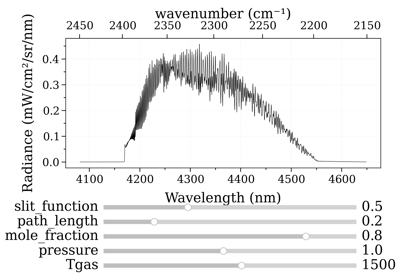Real-time GPU Accelerated Spectra (Interactive)

Real-time GPU Accelerated Spectra (Interactive)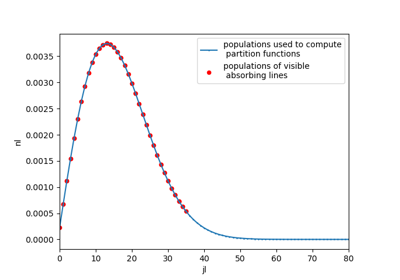See populations of computed levels

See populations of computed levels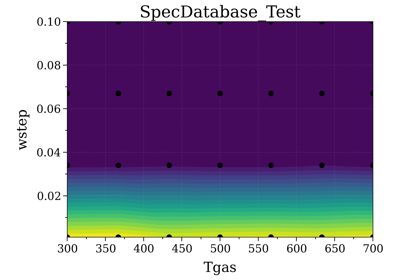Spectrum Database

Spectrum DatabaseCompare CO xsections from the ExoMol and HITEMP database

Compare CO xsections from the ExoMol and HITEMP database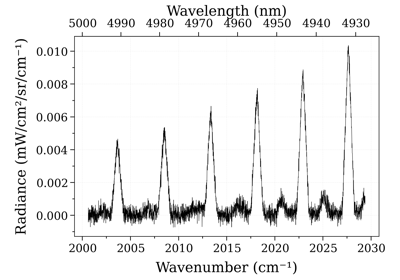Post-process using Specutils

Post-process using SpecutilsMulti-temperature Fit

Multi-temperature Fit1 temperature fit

1 temperature fitFit an equilibrium spectrum with Tgas as fit parameter using new fitting modules

Fit an equilibrium spectrum with Tgas as fit parameter using new fitting modulesFit a non-LTE spectrum with multiple fit parameters

Fit a non-LTE spectrum with multiple fit parameters

Refer to the guide below for an exhaustive list of all features:

For any other question you can use the Q&A forum, the GitHub issues or the community chats on Gitter or Slack .## How to generate a Spectrum?¶

### Calculate a Spectrum¶

Usually a Spectrum object is the output from a line-by-line (LBL) radiation code. Refer to the LBL documentation for that. Example using the RADIS LBL module with calc_spectrum():

from radis import calc_spectrum
s = calc_spectrum(...)        # s is a Spectrum object


Or with the SpectrumFactory class, which can be used to batch-generate multiple spectra using the same line database:

from radis import SpectrumFactory
sf = SpectrumFactory(...)
sf.fetch_databank("hitemp", load_columns='noneq')  # or 'hitran', 'exomol', etc.
s = sf.eq_spectrum(...)
s2 = sf.non_eq_spectrum(...)


Note that the SpectrumFactory class has the init_database() method that automatically retrieves a spectrum from a SpecDatabase if you calculated it already, or calculates it and stores it if you didn’t. Very useful for spectra that require long computational times!

### Initialize from Python arrays¶

The standard way to build a Radis Spectrum is from a dictionary of numpy arrays:

# w, k, I are numpy arrays for wavenumbers, absorption coefficient, and radiance.
from radis import Spectrum
s = Spectrum({"wavenumber":w, "abscoeff":k, "radiance_noslit":I},


Or:

s = Spectrum({"abscoeff":(w,k), "radiance_noslit":(w,I)},
wunit="cm-1"


You can also use the from_array() convenience function:

# w, T are two numpy arrays
from radis import Spectrum
s = Spectrum.from_array(w, T, 'transmittance_noslit',
wunit='nm', unit='') # adimensioned


Dimensionned arrays can also be used directly

import astropy.units as u
w = np.linspace(200, 300) * u.nm
I = np.random.rand(len(w)) * u.mW/u.cm**2/u.sr/u.nm
s = Spectrum.from_array(w, I, 'radiance_noslit')


Other convenience functions have been added to handle the usual cases: calculated_spectrum(), transmittance_spectrum() and experimental_spectrum():

# w, T, I are numpy arrays for wavelength, transmittance and radiance
from radis import calculated_spectrum, transmittance_spectrum, experimental_spectrum
s1 = calculated_spectrum(w, I, wunit='nm', Iunit='W/cm2/sr/nm')     # creates 'radiance_noslit'
s2 = transmittance_spectrum(w, T, wunit='nm')                       # creates 'transmittance_noslit'
s3 = experimental_spectrum(w, I, wunit='nm', Iunit='W/cm2/sr/nm')   # creates 'radiance'


### Initialize from Specutils¶

Use from_specutils() to convert from a specutils specutils.spectra.spectrum1d.Spectrum1D object

from radis import Spectrum
Spectrum.from_specutils(spectrum)


### Initialize from a text file¶

Spectrum objects can also be generated directly from a text file.

From a file, use from_txt()

# 'exp_spectrum.txt' contains a spectrum
from radis import Spectrum
s = Spectrum.from_txt('exp_spectrum.txt', 'radiance',
wunit='nm', unit='mW/cm2/sr/nm')


It is, however, recommended to use the RADIS .spec json format to store and load arrays :

### Load from a .spec file¶

A .spec file contains all the Spectrum spectral arrays as well as the input conditions used to generate it. To retrieve it use the load_spec() function:

s = load_spec('my_spectrum.spec')


Sometimes the .spec file may have been generated under a compressed format where redundant spectral arrays have been removed (for instance, transmittance if you already know absorbance). Use the update() method to regenerate missing spectral arrays:

s = load_spec('my_spectrum.spec', binary=True)
s.update()


If many spectra are stored in a folder, it may be time to set up a SpecDatabase structure to easily see all Spectrum conditions and get Spectrum that suits specific parametersLoad an experimental spectrum

Load an experimental spectrumRemove a baseline

Remove a baseline1 temperature fit

1 temperature fitFit an equilibrium spectrum with Tgas as fit parameter using new fitting modules

Fit an equilibrium spectrum with Tgas as fit parameter using new fitting modulesFit a non-LTE spectrum with multiple fit parameters

Fit a non-LTE spectrum with multiple fit parameters

### Load from a HDF5 file¶

This is the fastest way to read a Spectrum object from disk. It keeps metadata and units, and you can also load only a part of a very large spectrum. Use from_hdf5()

Spectrum.from_hdf5("rad_hdf.h5", wmin=2100, wmax=2200, columns=['abscoeff', 'emisscoeff'])


### Calculate a test spectrum¶

You need a spectrum immediatly, to run some tests ? Use test_spectrum()

s = radis.test_spectrum()
s.apply_slit(0.5, 'nm')


This returns the CO spectrum from the first documentation example

scale

60 %

### Generate a Blackbody (Planck) function object¶

In RADIS you can either use the planck() and planck_wn() functions that generate Planck radiation arrays for wavelength and wavenumber, respectively.

Or, you can use the sPlanck() function that returns a Spectrum object, with all the associated methods (add in a line-of-sight, compare, etc.)

Example:

s = sPlanck(wavelength_min=3000, wavelength_max=50000,
T=288, eps=1)
s.plot()## Spectral Arrays¶

A Spectrum object can contain one spectral arrays, such as 'radiance' for emission spectra, or 'transmittance' for absorption spectra. It can also contain both emission and absorption quantities to be later combined with other spectra by solving the radiative transfer equation.

Some variables represent quantities that have been convolved with an instrumental slit function, as measured in experimental spectra:

• 'radiance': the spectral radiance, convolved by the instrument function (typically in :math:'mW/cm^2/sr/nm'). This is sometimes confusingly called spectral intensity.

• 'transmittance': the directional spectral transmittance (:math:0 to :math:1), convolved by the instrument function.

• 'emissivity': the directional spectral emissivity (:math:0 to :math:1), convolved by the instrument function. The spectral emissivity is the radiance emitted by a surface divided by that emitted by a black body at the same temperature as that surface. This value is only defined under thermal equilibrium.

Other variables represent quantities that have not been convolved (theoretical spectra):

• 'radiance_noslit': the spectral radiance (typically in $$mW/cm^2/sr/nm$$). This

is sometimes confusingly called spectral intensity. - 'transmittance_noslit': the directional spectral transmittance (:math:0 to :math:1) - 'emissivity_noslit': spectral emissivity (0 to 1) i.e. the radiance emitted by a surface

divided by that emitted by a black body at the same temperature as that surface. This value is only defined under thermal equilibrium.

• 'emisscoeff': the directional spectral emission density (typically in :math:'mW/cm^3/sr/nm').

• 'absorbance': the directional spectral absorbance (no dimension), also called optical depth.

• 'abscoeff': spectral absorption coefficient (typically in :math:'cm^{-1}'), also called opacity. This is sometimes found as the extinction coefficient in the literature (strictly speaking, extinction is absorption + diffusion, the latter being negligible in the infrared).

• 'xsection': absorption cross-section, typically in cm2

Additionally, RADIS may calculate extra quantities such as:

• 'emisscoeff_continuum': the pseudo-continuum part of the spectral emission density 'emisscoeff', that can be generated by SpectrumFactory

• 'abscoeff_continuum' the pseudo-continuum part of the spectral absorption coefficient 'abscoeff', that can be generated by SpectrumFactory

See the latest list in the CONVOLUTED_QUANTITIES and NON_CONVOLUTED_QUANTITIES.

### Custom spectral arrays¶

A Spectrum object is built on top of a dictionary structure, and can handle spectral arrays with any name.

Custom spectral arrays with arbitrary units can be defined when creating a Spectrum object, for instance:

# w, I are two numpy arrays
s = Spectrum.from_array(w, I, 'irradiance',
wunit='nm', unit='w/cm2/nm')


Although not recommended, it is also possible to directly edit the dictionary containing the objects. For instance, this is done in CO2 radiative forcing example to calculate irradiance from radiance (by multiplying by 'pi' and changing the unit):

s._q['irradiance'] = s.get('radiance_noslit')*pi


The unit conversion methods will properly work with custom units.

Warning

Rescaling or combining spectra with custom quantities may result in errors.

### Relations between quantities¶

Most of the quantities above can be recomputed from one another. In a homogeneous slab, one requires an emission spectral density, and an absorption spectral density, to be able to recompute the other quantities (provided that conditions such as path length are given). Under equilibrium, only one quantity is needed. Missing quantities can be recomputed automatically with the update() method.

### Units¶

Default units are stored in the units dictionary

It is strongly advised not to modify the dictionary above. However, spectral arrays can be retrieved in arbitrary units with the get() method.

When a spectral unit is convolved with apply_slit(), a new convolved spectral array is created. The unit of the convolved spectral array may be different, depending on how the slit function was normalized. Several options are available in RADIS. Please refer to the documentation of the apply_slit() method.

## How to access Spectrum properties?¶

### Get spectral arrays¶

Spectral Arrays of a Spectrum object can be stored in arbitrary wavespace (wavenumbers, wavelengths in air, or wavelengths in vacuum) and arbitrary units.

Therefore, it is recommendeded to use the get() method to retrieve the quantity un the units you want:

w, I = s.get('transmittance_noslit', wunit='cm-1')
_, R = s.get('radiance_noslit', wunit='nm', Iunit='W/cm2/sr/nm',
medium='air')


Use with return_units to get dimensioned Astropy Quantities

w, R  = s.get('radiance_noslit', return_units=True)
# w, R are astropy quantities


See spectral arrays for the list of spectral arrays.

### Get wavelength/wavenumber¶

Use the get_wavelength() and get_wavenumber() methods:

w_nm = s.get_wavelength()
w_cm = s.get_wavenumber()


### Plot spectral arrays¶

Use plot():

s.plot('radiance_noslit')


You can plot on the same figure as before using the convenient nfig parameter:

s_exp.plot('radiance_noslit', nfig='same')


But for comparing different spectra you may want to use plot_diff() directly.

### Plot populations¶

Get or plot populations computed in calculations. Use get_populations() or plot_populations():

s.plot_populations('vib', nunit='cm-3')See populations of computed levels

See populations of computed levels

### Plot line survey¶

Use the line_survey() method. Example of output:

from radis import SpectrumFactory
sf = SpectrumFactory(
wavenum_min=2380,
wavenum_max=2400,
mole_fraction=400e-6,
path_length=100,  # cm
isotope=,
)
s = sf.eq_spectrum(Tgas=1500)
s.apply_slit(0.5)Line Survey

Line SurveySee populations of computed levels

See populations of computed levels

### Know if a spectrum has nan¶

has_nan() looks over all spectral quantities. print(s) will also show the number of nans per quantity

s = radis.test_spectrum()
s.has_nan()


## How to export ?¶

### Save a Spectrum object¶

To store use the store() method:

# s is a Spectrum object
s.store('temp_file.spec')
assert s == s2  # compare both


The generated .spec file can be read (and edited) with any text editor. However, it may take a lot of space. If memory is important, you may use the compress=True argument which will remove redundant spectral arrays (for instance, transmittance if you already know absorbance), and store the .spec file under binary format. Use the update() method to regenerate missing quantities:

s.store('temp_file.spec', compress=True, if_exists_then='replace')
s2.update()    # regenerate missing quantities


If calculating many spectra, you may want to handle all of them together in a SpecDatabase. You can add them to the existing database with the add() method:

db = SpecDatabase(r"path/to/database")     # create or loads database


Note that if using the RADIS LBL code to generate your spectra, the SpectrumFactory class has the init_database() method that automatically retrieves a spectrum from a database if you calculated it already, or calculates it and stores it if you didn’t. Very useful for spectra that requires long computational times!

### Export to hdf5¶

This is the fastest way to dump a Spectrum object on disk (and also, it keeps metadata and therefore units !). Use to_hdf5()

s.to_hdf5('spec01.h5')


### Export to txt¶

Saving to .txt in general isn’t recommended as you will loose some information (for instance, the conditions). You better use store() and export to .spec [a hidden .json] format.

If you really need to export a given spectral arrays to txt file (for use in another software, for instance), you can use the savetxt() method that will export a given spectral arrays:

s.savetxt('radiance_W_cm2_sr_um.csv', 'radiance_noslit', wunit='nm', Iunit='W/cm2/sr/µm')


### Export to Pandas¶

s.to_pandas()


This will return a DataFrame with all spectral arrays as columns.

### Export to Specutils¶

Use to_specutils() to convert to a to specutils specutils.spectra.spectrum1d.Spectrum1D object

s.to_specutils()Post-process using Specutils

Post-process using Specutils

## How to modify a Spectrum object?¶

### Calculate missing quantities¶

Some spectral arrays can be infered from quantities stored in the Spectrum if enough conditions are given. For instance, transmittance can be recomputed from the spectral absorption coefficient if the path length is stored in the conditions.

The update() method can be used to do that. In the example below, we recompute transmittance from the absorption coefficient (opacity)

# w, A are numpy arrays for wavenumber and absorption coefficient
s = Spectrum.from_array(w, A, 'abscoeff', wunit='cm-1')
s.update('transmittance_noslit')


All derivable quantities can be computed using .update('all') or simply .update():

s.update()


### Update Spectrum conditions¶

Spectrum conditions are stored in a conditions dictionary

Conditions can be updated a posteriori by modifying the dictionary:

s.conditions['path_length'] = 10    # cm


### Rescale Spectrum with new path length¶

Path length can be changed after the spectra were calculated with the rescale_path_length() method. If the spectrum is not optically thin, this requires solving the radiative transfer equation again, so the emisscoeff and abscoeff (opacity) quantities will have to be stored in the Spectrum, or any equivalent combination (radiance_noslit and absorbance, for instance).

Example:

from radis import load_spec
s.rescale_path_length(0.5)      # calculate for new path_length


### Rescale Spectrum with new mole fraction¶

Warning

Rescaling mole fractions neglects the changes in collisional broadening

mole fraction can also be changed in post-processing, using the rescale_mole_fraction() method that works similarly to the rescale_path_length() method. However, the broadening coefficients are left unchanged, which is valid for small mole fraction changes. However, for large mole fraction changes you will have to recalculate the spectrum from scratch.

>>> s.rescale_mole_fraction(0.02)   # calculate for new mole fraction


### Apply instrumental slit function¶

s.apply_slit(1.5)    # nm


By default, convoluted spectra are thinner than non-convoluted spectra, to remove side effects. Use the mode= argument to change this behavior.Line Survey

Line SurveyGPU Accelerated Spectra

GPU Accelerated SpectraCalculate non-LTE spectra of carbon-monoxide

Calculate non-LTE spectra of carbon-monoxideCalculate a spectrum from ExoMol

Calculate a spectrum from ExoMol

### Plot the slit function that was applied¶

Use plot_slit(). You can also change the unit:

s.apply_slit(0.5, 'cm-1')    # for instance
s.plot_slit('nm')


### Multiply, subtract¶

Sometimes you need to manipulate an experimental spectrum, to account for calibration or remove a baseline. Spectrum operations are done just for that:

Most of these functions are implemented with the standard operators. Ex:

((s_exp - 0.1)*10).plot()   # works for a Spectrum s_exp


Note that these operators are purely algebraic and should not be used in place of the line-of-sight functions, i.e, SerialSlabs() (>) and MergeSlabs() (//)

Most of these functions will only work if there is only one spectral arrays defined in the Spectrum. If there is any ambiguity, use the take() method. For instance, the following line is a valid RADIS command to plot the spectral radiance of a spectrum with a low resolution:

(10*(s.apply_slit(10, 'nm')).take('radiance')).plot()


Algebraic operations also work with dimensioned Quantity. For instance, remove a constant baseline in a given unit:

s -= 0.1 * u.Unit('W/cm2/sr/nm')


The max() function returns a dimensionned value, therefore it can be used to normalize a spectrum directly :

s /= s.max()


Or below, we calibrate a Spectrum, assuming the spectrum units is in “count”, and that our calibration show we have 94 $$mW/cm2/sr/nm$$ per count.

s *= 94 * u.Unit("mW/cm2/sr/nm") / u.Unit("count")


### Offset, crop¶

Use the associated functions: crop(), offset().

They are also defined as methods of the Spectrum objects (see crop(), offset()), so they can be used directly with:

s.offset(3, 'nm')
s.crop(370, 380, 'nm')


By default, using methods that will modify the object in place, using the functions will generate a new Spectrum.Load an experimental spectrum

Load an experimental spectrum

### Normalize¶

Use normalize() directly, if your spectrum only has one spectral arrays

s.normalize()
s.normalize(normalize_how="max")
s.normalize(normalize_how="area")


You can also normalize only on limited range. Useful for noisy spectra

s.normalize(wrange=(2250, 2500), wunit="cm-1", normalize_how="mean")


This returns a new spectrum and does not modify the Spectrum itself. To do so use:

s.normalize(inplace=True)


### Chaining¶

You can chain the various methods of Spectrum. For instance:

s.normalize().plot()


Or:

s.crop(4120, 4220, 'nm').apply_slit(3, 'nm').take('radiance')


If you want to create a new spectrum, don’t forget to set inplace=False for the first command that allows it. i.e

s2 = s.crop(4120, 4220, 'nm', inplace=False).apply_slit(3, 'nm').offset(1.5, 'nm')


### Remove a baseline¶

Either use the add_constant() mentionned above, which is implemented with the - operator:

s2 = s - 0.1


Or remove a linear baseline with:

You could also use the functions available in pyspecutils, see to_specutils().

### Calculate transmittance from radiance with Kirchoff’s law¶

RADIS can be used to infer spectral arrays from others if they can be derived. If on top that, equilibrium is assumed, then Kirchoff’s law is used. See How to ... calculate missing quantities? and the update() method with argument assume_equilibrium=True. Example:

s = calculated_spectrum(...)     # defines 'radiance_noslit')
s.update('transmittance_noslit')
s.plot('transmittance_noslit')


You can infer if a Spectrum is at (thermal) equilibrium with the is_at_equilibrium() method, that looks up the declared spectrum conditions and ensures Tgas==Tvib==Trot. It does not imply chemical equilibrium (mole fractions are still arbitrary)

## How to handle multiple Spectra?¶

### Build a line-of-sight profile¶

RADIS allows the combination of Spectra such as:

s_line_of_sight = (s_plasma_CO2 // s_plasma_CO) > (s_room_absorption)


Refer to the line-of-sight module

### Compare two Spectra¶

You can compare two Spectrum objects using the compare_with() method, or simply the == statement (which is essentially the same thing):

s1 == s2
>>> True/False
s1.compare_with(s2)
>>> True/False


However, compare_with() allows more freedom regarding what quantities to compare. == will compare everything of two spectra, including input conditions, units under which spectral quantities are stored, populations of species if they were saved, etc. In many situations, we may want to simply compare the spectra themselves, or even a particular quantity like transmittance_noslit. Use:

s1.compare_with(s2, spectra_only=True)                    # compares all spectral arrays
s1.compare_with(s2, spectra_only='transmittance_noslit')  # compares transmittance only


The aforementionned methods will return a boolean array (True/False). If you need the difference, or ratio, or distance, between your two spectra, or simply want to plot the difference, you can use one of the predefined functions get_diff(), get_ratio(), get_distance(), get_residual() or the plot function plot_diff():

from radis.spectrum import plot_diff


These functions usually require that the spectra are calculated on the same spectral range. When comparing, let’s say, a calculated spectrum with experimental data, you may want to interpolate: you can have a look at the resample() method. See Interpolate a Spectrum on another for details.

In plot_diff(), you can choose to plot the absolute difference (method='diff'), or the ratio (method='ratio'), or both:

# Below we compare 2 CO2 spectra s_cdsd and s_hitemp previously calculated with two different line databases.
from radis import plot_diff
plot_diff(s_cdsd, s_hitemp, method=['diff', 'ratio'])### Plot in log scale¶

If you wish to plot in a logscale, it can be done in the following way:’:

fig, [ax0, ax1] = plot_diff(s_expe, s_test, normalize=False, verbose=False)
ylim0 = ax0.get_ybound()
ax0.set_yscale("log")
ax0.set_ybound(ylim0)


### Fit an experimental spectrum¶

RADIS does not include fitting algorithms. To fit an experimental spectrum, one should use one of the widely available optimization algorithms from the Python ecosystem, for instance scipy.optimize.minimize().

The get_residual() and get_residual_integral() functions can be used to return a scalar to feed to the minimize() function.

A simple fitting procedure could be:

from scipy.optimize import minimize
from radis import calc_spectrum, experimental_spectrum

s_exp = experimental_spectrum(...)

def cost_function(T):
calc_spectrum(Tgas=T,
... # other parameters )
return get_residual(s_exp, s)

best = minimize(cost_function,
800, # initial value
bounds=[500, 2000],
)
T_best = best.x


Note however that the performances of a fitting procedure can be vastly improved by not reloading the line database every time. In that case, it becomes interesting to use the SpectrumFactory class.

An example of a script that uses the SpectrumFactory, multiple fitting parameters, and plots the residual and the calculated spectrum in real-time, can be found in the Examples page

### Interpolate a Spectrum on another¶

Let’s interpolate a calculated spectrum on an experimental spectrum, using the resample() and, for instance, the get_wavelength() method:

# let's say we have two objects:
s_calc = calc_spectrum(...)
# resample:
s_calc.resample(s_exp.get_wavelength(), 'nm')


Energy conservation is ensured and an error is raised if your interpolation is too bad. If you need to adjust the error threshold, see the parameters in resample().

### Create a database of Spectrum objects¶

Use the SpecDatabase class. It takes a folder as an argument, and converts it in a list of Spectrum objects. Then, you can:

## Spectrum Database¶

Spectrum objects can be stored/loaded to/from .spec JSON files using the store() method and the load_spec() function.Spectrum Database

Spectrum Database

It is also possible to set up a SpecDatabase which reads all .spec files in a folder. The SpecDatabase can then be connected to a SpectrumFactory so that spectra already in the database are not recomputed, and that new calculated spectra are stored in the folder

Example:

db = SpecDatabase(r"path/to/database")     # create or loads database

db.update()  # in case something changed
db.see(['Tvib', 'Trot'])   # nice print in console

s = db.get('Tvib==3000')  # get a Spectrum back
db.add(s)  # update database (and raise error because duplicate!)


A SpecDatabase can also be used to compare the physical and computation parameters of all spectra in a folder. Indeed, whenever the database is loaded, a summary .csv file is generated that contains all conditions and can be read, for instance, with Excel.

Example:

from radis import SpecDatabase
SpecDatabase(r".")     # this generates a .csv file in the current folder


The examples below show some actions that can be performed on a database:

### Iterate over all Spectra in a database¶

Both methods below are equivalent. Directly iterating over the database:

db = SpecDatabase('.')
for s in db:
print(s.name)


Or using the get() method with no filtering condition:

db = SpecDatabase('.')
for s in db.get():
print(s.name)


You can also use dictionary-like methods: keys(), values() and items() where Spectrum are returned under a {path:Spectrum} dictionary.

### Filter spectra that match certain conditions¶

If you want to get Spectra in your database that match certain conditions (e.g: a particular temperature), you may want to have a look at the get(), get_unique() and get_closest() methods

### Fit an experimental spectrum against precomputed spectra¶

The fit_spectrum() method of SpecDatabase can be used to return the spectrum of the database that matches the best an experimental spectrum:

s_exp = experimental_spectrum(...)
db = SpecDatabase('...')
db.fit_spectrum(s_exp)


By default fit_spectrum() uses the get_residual() function. You can use an customized function too (below: to get the transmittance):

from radis import get_residual
db.fit_spectrum(s_exp, get_residual=lambda s_exp, s: get_residual(s_exp, s, var='transmittance'))


You don’t necessarily need to precompute spectra to fit an experimental spectrum. You can find an example of multi temperature fitting script in the Example pages, which shows the evolution of the spectra in real-time. You can get inspiration from there!

### Updating a database¶

Update all spectra in current folder with a new condition (‘author’), making use of the items() method:

from radis import SpecDatabase
db = SpecDatabase('.')
for path, s in db.items():
s.conditions['author'] = 'me'
s.store(path, if_exists_then='replace')


You may also be interested in the map() method.

### When not to use a Database¶

If you simply want to store and reload one Spectrum object, no need to use a database: you better use the store() method and load_spec() function.

Databases prove useful only when you want to filter precomputed Spectra based on certain conditions.